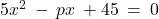## For what values of p will the equation 5×2 – px +45 = 0 have equal roots​

Question

For what values of p will the equation 5×2 – px +45 = 0 have equal roots​

in progress 0
5 months 2021-08-04T20:08:57+00:00 1 Answers 10 views 0

The two solutions are:

p = 30 and p = -30

Step-by-step explanation:

Given the equation• We know that the quadratic equation ax²+bx+c=0 will have equal roots if its discriminant b²-4ac = 0a=5, b=-p, c=45

b²-4ac = 0

(-p)² – 4(5)(45)=0

p²-900=0

p² = 900

p = ± 30

The two solutions are:

p = 30 and p = -30